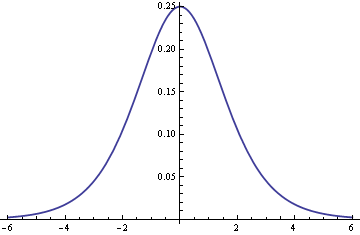# Normal approximation to logistic distribution

The logistic distribution looks very much like a normal distribution. Here’s a plot of the density for a logistic distribution.This suggests we could approximate a logistic distribution by a normal distribution. (Or the other way around: sometimes it would be handy to approximate a normal distribution by a logistic. Always invert.)

But which normal distribution approximates a logistic distribution? That is, how should we pick the variance of the normal distribution?

The logistic distribution is most easily described by its distribution function (CDF):

F(x) = exp(x) / (1 + exp(x)).

To find the density (PDF), just differentiate the expression above. You can add a location and scale parameter, but we’ll keep it simple and assume the location (mean) is 0 and the scale is 1.  Since the logistic distribution is symmetric about 0, we set the mean of the normal to 0 so it is also symmetric about 0. But how should we pick the scale (standard deviation) σ for the normal?

The most natural thing to do, or at least the easiest thing to do, is to match moments: pick the variance of the normal so that both distributions have the same variance. This says we should use a normal with variance σ2 = π2/3 or σ = π/√3 . How well does that work? The following graph gives the answer. The logistic density is given by the solid blue line and the normal density is given by the dashed orange line.Not bad, but we could do better. We could search for the value of σ that minimizes the difference between the two densities. The minimum occurs around σ = 1.6. Here is the revised graph using that value.The maximum difference is almost three times smaller when we use σ = 1.6 rather than σ = π/√ 3 ≈ 1.8.

What if we want to minimize the difference between the distribution (CDF) functions rather than the density (PDF) functions? It turns out we end up at about the same spot: set σ to approximately 1.6. The two optimization problems don’t have exactly the same solution, but the two solutions are close.

The maximum difference between the distribution function of a logistic and the distribution of a normal with σ = 1.6 is about 0.017. If we used moment matching and set σ = π/√3, the maximum difference would be about 0.022. So moment matching does a better job of approximating the CDFs than approximating the PDFs. But we don’t need to decide between the two criteria since setting σ = 1.6 approximately minimizes both measures of the approximation.

## 12 thoughts on “Normal approximation to logistic distribution”

1. Dj Gilcrease

wonder if σ = (1 + √5)/2 minimizes both, doubt it but would be an interesting coincidence if it did

2. Jurgen

Cool! Turns out the moment matching solution is also the solution of finding the standard deviation of the normal distribution which minimizes the KL-divergence: min_{sigma} KL(Logistic(x)||Normal(x;0,sigma)). I have a vague suspicion that the one which minimizes the density difference is the minimum of min_{sigma} KL(Normal(x;0,sigma)||Logistic(x)) but haven’t checked this …

3. Am struggling with concept of “Distribution of Functions of Random Variables”.

What is Logistics Distribution? How to apply it? Any quick help will be appreciated.

4. any logistic regression implementation ;) thank u

5. Hi, John, thanks for your post! I have a simple question: what is the difference between normal distribution and logistic distribution? I mean, I know them have different formulation, but in real case like logistic regression, why do we have to choose logistic distribution, if we choose normal distribution, what will happen? Furthermore, in what case should we use logistic distribution and in what case we should use the normal distribution.

6. ct: One reason for using logistic regression, i.e. using logit for a link function, is that it falls naturally out of the representation of a binomial distribution as a member of the exponential family.

However, some people do use a normal distribution, i.e. using the probit link function. I don’t know what the advantages of this approach are.

7. KH Kim

Theoretically, if we assume some latent error,
it’s natural to think of it having normal distribution.
So the normal distribution, like other error distribution

8. David C

Great post. I just want to add that this approximation is great not too far from the mean, that is about less than about 2 std dev. But if you plan to work with rare events, the approximation is getting poor (the normal distribution assigns rare events a much lower probability than the logistic one). That can be seen when plotting the densities on the log scale (for the y-axis).

Great post. I just want add another difference. I would prefer to use logistic distribution because its CDF is available in closed form solution, whereas it is not the case in guassian/normal distribution.

10. David W Locke

So we approximated the Poisson via the normal, and now we are approximating the logistic via the normal. I tend to work in the other direction. I approximate the normal from the Poisson because I can approximate a normal from the Poisson a long time before I have achieved normality.

Approximating other distributions from the normal assumes you have a normal. This problem is well hidden by the use of dataset, rather than data. Yes, “rather than.” If we used data that had not yet achieved normality, these nice symmetric figures wouldn’t be seen as realistic.

Don’t assume normality.

11. David W Locke

Do the normal distribution and logistic regressions achieve normality at lower n values?